Online interactive resources for the Scottish Maths curriculum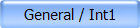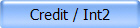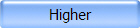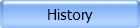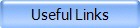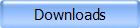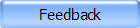Advanced Higher Madras College Advanced Higher Links Covering Units 1-3 S-Cool! - A/AS Level Revision Revision topics include those applicable to Advanced Higher Maths. Algebraic Long Division (Java Applet) Examples showing full working. Division by linear function only. Calculus 1 Step by step differentiation & integration Enter a function and see the step by step solution. Includes chain, product and quotient rules. Calculus 3 (Flash) Who Doesn't Want To Be A Millionaire Test your knowledge of differentiation & integration.Complex Numbers (Java Applet) Argand Diagram Display, add, subtract, multiply and divide two complex numbers. Cartesian and modulus-argument form given. Matrices (Flash) Animated examples of matrix operations. Add, subtract, multiply by a scalar/matrix to up to 5x5 matrices. Equation Solver Solves three equations in three unknowns. Euclidian Algorithm (Java Applet) Computes the greatest common divisor of two given integers via the Euclidean Algorithm. Shows all the steps. Vectors 1 (Java Applet) On-line help with 3D vectors. Includes some Java Applets. Vectors 2 (Java Applet) The Vector Cross Product - an interactive tutorial. Vectors 3 (Java Applet) Vector Equation of a Line in Three-Dimensional Space Input two points to obtain the vector equation of the line through them and see it drawn on 3D axes. Newtons Method 1 (Java Applet) Newtons Method 2) (Java Applet)